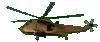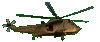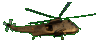Common Factor of a Trinomial       Fun Game Tips:

- This game provides practice in finding a factor which is common to all three terms.

- Example 1: The trinomial expression (3w+6t-3) has the common factor (3).
When you factor the trinomial (3w+6t-3), the answer is   3w+6t-3 = 3(w+2t-1).

- Example 2: The trinomial expression (12w2+3w-6wt) has the common factor (3w).
When you factor the trinomial (12w2+3w-6wt), the answer is    12w2+3w-6wt = 3w(4w+1-2t).
Check your answer by distributing the factor (3w) times the factor (4w+1-2t) to get (12w2+3w-6wt) .

- Your Game Score is reduced by the number of helicopter hits.

- To slow the game speed repeat tap/click on the word Slider.
- To increase the game speed repeat tap/click on the word Math.
- Speed can also be adjusted with a keyboard's - and + keys.

- Refresh/Reload the web page to restart the game.